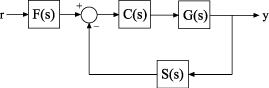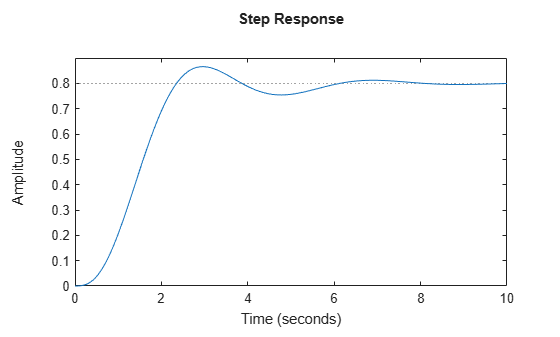# Control System Modeling with Model Objects

Model objects can represent individual components of a control architecture, such as the plant, actuators, sensors, or controllers. You can connect model objects to build aggregate models of block diagrams that represent the combined response of multiple elements.

For example, the following control system contains a prefilter F, a plant G, and a controller C, arranged in a single-loop configuration. The model also includes a representation of sensor dynamics, S.You can represent each of the components as a model object. You do not need to use the same type of model object for each component. For example, represent the plant G as a zero-pole-gain (`zpk`) model with a double pole at `s` = -1; C as a PID controller, and F and S as transfer functions:

```G = zpk([],[-1,-1],1); C = pid(2,1.3,0.3,0.5); S = tf(5,[1 4]); F = tf(1,[1 1]);```

You can then combine these elements build models that represent your control system or the control system as a whole. For example, create the open-loop response SGC:

`open_loop = S*G*C;`

To build a model of the unfiltered closed-loop response, use the `feedback` command:

`T = feedback(G*C,S);`

To model the entire closed-loop system response from r to y, combine T with the filter transfer function:

`Try = T*F;`

The results `open_loop`, `T`, and `Try` are also linear model objects. You can operate on them with Control System Toolbox™ control design and analysis commands. For example, plot the step response of the entire system:

`stepplot(Try)`When you combine Numeric LTI models, the resulting Numeric LTI model represents the aggregate system. The resulting model does not retain the original data from the combined components. For example, `T` does not separately keep track of the dynamics of the components `G`, `C`, and `S` that are combined to create `T`.